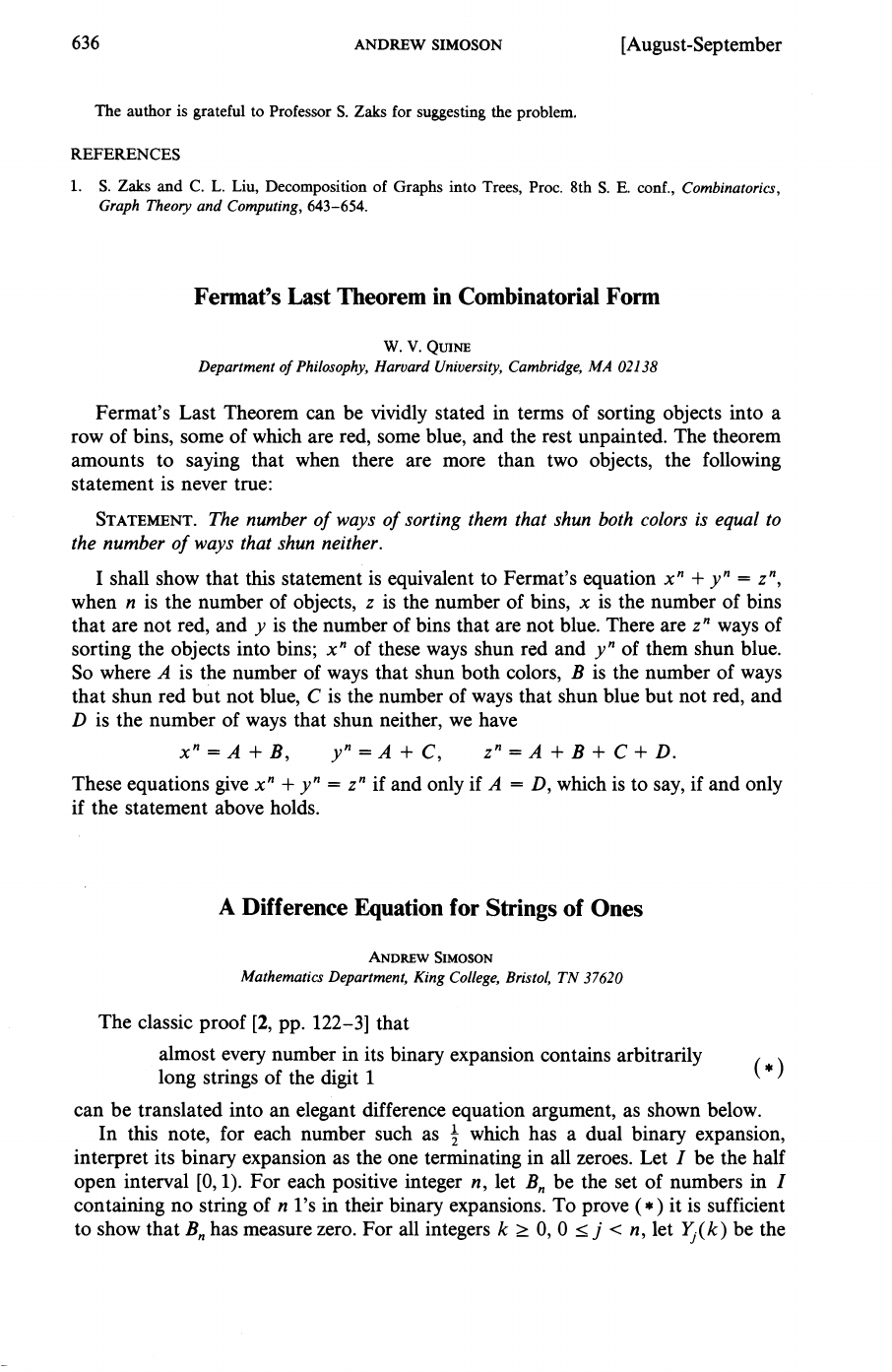Willard Van Orman Quine was one of the most influential philosopher...
W.V. Quine came up with a different interpretation for Fermat's Las...
If you have $n$ indistinguishable balls and $z$ bins, each ball has...636
ANDREW
SIMOSON
[August-September
The author is grateful to Professor
S.
Zaks for suggesting the problem.
REFERENCES
1.
S.
Zaks
and
C.
L.
Liu, Decomposition of Graphs into Trees, Proc. 8th
S. E.
conf.,
Combinatorics,
Graph Theo~ and Computing,
643-654.
Fermat's Last Theorem in Combinatorial Form
W.
V.
QUNE
Department of Philosophy, Harvard University, Cambridge,
MA
02138
Fermat's Last Theorem can be vividly stated in terms of sorting objects into a
row of bins, some of which are red, some blue, and the rest unpainted. The theorem
amounts to saying that when there are more than two objects, the following
statement is never true:
STATEMENT.The number of ways of sorting them that shun both colors is equal to
the number of ways that shun neither.
I shall show that this statement is equivalent to Fermat's equation xn
+
yn
=
zn,
when n is the number of objects, z is the number of bins, x is the number of bins
that are not red, and y is the number of bins that are not blue. There are zn ways of
sorting the objects into bins; xn of these ways shun red and yn of them shun blue.
So where A is the number of ways that shun both colors, B is the number of ways
that shun red but not blue, C is the number of ways that shun blue but not red, and
D is the number of ways that shun neither, we have
xn=A+B, yn=A+C, zn=A+B+C+D.
These equations give xn
+
yn
=
zn if and only if A
=
D, which is to say, if and only
if the statement above holds.
A
Difference Equation for Strings of Ones
ANDREWSIMOSON
Mathematics Department, King College, Bristol,
TN
37620
The classic proof
[2,
pp. 122-31 that
almost every number in its binary expansion contains arbitrarily
long strings of the digit
1
(*
>
can be translated into an elegant difference equation argument, as shown below.
In this note, for each number such as which has a dual binary expansion,
interpret its binary expansion as the one terminating in all zeroes. Let
I
be the half
open interval [0, 1). For each positive integer n, let Bn be the set of numbers in
I
containing no string of n 1's in their binary expansions. To prove
(*)
it is sufficient
to show that Bn has measure zero. For all integers k
2
0, 0
I
j
<
n, let Y,(k) be the
Willard Van Orman Quine was one of the most influential philosophers of the twentieth century as well as a respected logician. He wrote this paper while teaching at Harvard University where he spent all his career in one way or another, first as a student, then as a professor of philosophy and a teacher of logic and set theory, and finally as a professor emeritus who published or revised several books in retirement. ![](http://news.harvard.edu/gazette/2001/01.18/photos/09-vanquine-325.jpg) If you have $n$ indistinguishable balls and $z$ bins, each ball has $z$ possibilities to choose in terms of bins and since we have $n$ balls the total number of ways to sort them is $\overbrace{z.z.z...z}^\text{n times} = z^n$ ![](http://i.imgur.com/H0Go9eC.png) Applying the same kind of reasoning, it is not difficult to see that $x^n$ and $y^n$ are the total number of ways to sort the balls avoiding red and blue bins respectively. Now, if we only have blue and unpainted bins there are just 2 ways of sorting the balls: either all balls end up in unpainted bins (A), or at least one gets into a blue bin (B). As a consequence: $x^n = A + B$ The same applies for $y^n = A +C$, where C is the case when at least one ball gets into a red bin. The total number of ways to sort the balls $z^n$ include cases A,B,C and finally D - when balls get into blue and red bins: $z^n = A +B+C+D$. Finally $x^n +y^n = z^n$ if and only if $D=A$. W.V. Quine came up with a different interpretation for Fermat's Last Theorem that is based purely in combinatorial arguments. Fermat's Last Theorem states that no three positive integers $x, y,$ and $z$ satisfy the equation $x^n + y^n = z^n$ for any integer value of $n>2$. There are several interpretations of FLT. One of the most famous is the geometrical interpretation where the equivalent proposition is: the sum of the volumes of two distinct n-dimensional cubes, whose lengths of the edges are respectively $x$ and $y$, is not equal to the volume of a third n-dimensional cube, whose length of the edge is $z$. ![](http://i.imgur.com/IP15zMU.png)